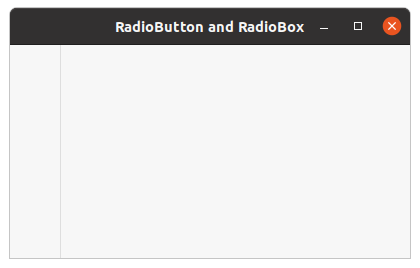Related Articles
wxPython – GetDefaultSize() method in wx.StaticLine
• Last Updated : 08 Jul, 2020

In this article we are going to learn about method GetDefaultSize() associated with wx.StaticLine class of wxPython. GetDefaultSize() method is simply used to return the size which will be given to the smaller dimension of the static line, i.e.

Its height for a horizontal line or its width for a vertical one.

Syntax: wx.StaticLine.GetDefaultSize()

Parameters: No parameters are required in GetDefaultSize() method.

Return Type: int

Code Example:

 `import` `wx`` ` ` ` `class` `FrameUI(wx.Frame):`` ` `    ``def` `__init__(``self``, parent, title):``        ``super``(FrameUI, ``self``).__init__(parent, title ``=` `title, size ``=``(``300``, ``200``))`` ` `        ``# function for in-frame components``        ``self``.InitUI()`` ` `    ``def` `InitUI(``self``):``        ``# parent panel for radio box``        ``pnl ``=` `wx.Panel(``self``)`` ` `        ``# list of choices``        ``hlist ``=` `[``'Item One'``, ``'Item Two'``]``        ``vlist ``=``[``'Item One'``, ``'Item Two'``]`` ` `        ``# create vertical line from point (50, 0) t0 (50, 250)``        ``self``.sl ``=` `wx.StaticLine(pnl, ``2``,  pos ``=``(``50``, ``0``), size ``=` `(``1``, ``250``),  ``                                              ``style ``=` `wx.LI_VERTICAL)`` ` `        ``# print size of the smaller dimension of static line``        ``print` `(``self``.sl.GetDefaultSize())`` ` `        ``# set frame in centre``        ``self``.Centre()``        ``# set size of frame``        ``self``.SetSize((``400``, ``250``))``        ``# show output frame``        ``self``.Show(``True``)`` ` ` ` ` ` `# wx App instance``ex ``=` `wx.App()``# Example instance``FrameUI(``None``, ``'RadioButton and RadioBox'``)``ex.MainLoop()`

Console Output:

```2
```

Output Window:Attention geek! Strengthen your foundations with the Python Programming Foundation Course and learn the basics.

To begin with, your interview preparations Enhance your Data Structures concepts with the Python DS Course. And to begin with your Machine Learning Journey, join the Machine Learning – Basic Level Course

My Personal Notes arrow_drop_up